## Precalculus Solver for the TiNspire CX

Need help with Precalculus homework? You’re in the right place! Our Precalculus solver app will be your best friend. This app will step by step solve your Precalculus problems immediately. Check out our Precalculus solver video below and try out the Precalculus trial at www.tinspireapps.com

Posted on Categories preCalculus, solver, tinspirecx

## ▷[Solved] Compute Limits in Calculus – Step by Step – using the TiNspire CX CAS

In your Calculus class you will be asked to compute limits. You should know that there is a variety of techniques to figure out limits such as via Factoring, via Common Denominator and of course via L’Hopital rule (which we covered in earlier posts already) . All these techniques can be applied using the Calculus Made Easy app , just navigate to Limits in the Menu as seen below:When selecting the menu option 4: Limit via Factoring you enter your function and the x value that you are approaching. Now lean back and observe the steps to arrive at the limit. Calculus all of a sudden got easy! See below.Another common method to compute Limits is via Common Denominator of the numerator. Read the sentence again to digest it better 😉

The tricky part is to enter the two fractions in the numerator properly, extra set of parentheses are needed here. See belowThe steps to compute the limits are displayed below: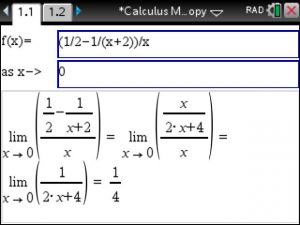These Step by Step Limit computation methods using your TiNspire CX CAS will allow you to master your Calculus class.

Posted on Categories calculus, Limits, solver, tinspirecx

## ▷Matrix Solver for the TiNspire : Solve any Matrix Equation using the TiNspire CX CAS and Matrix Made Easy

Using Matrix Made Easy at https://www.tinspireapps.com/?a=MME

Solve any Matrix Equation like the ones below: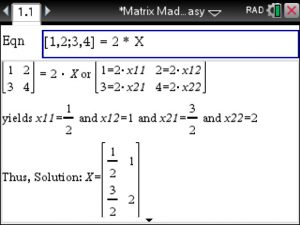Another example: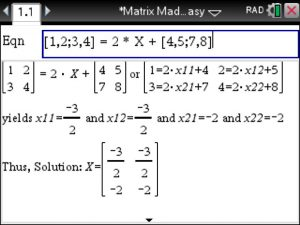## VIDEO: Solve 2×2 System of Equations in Algebra using the TiNspire – Step by Step

Here is a brief video showing how to solve any 2×2 system of equations in Algebra using the TiNspire calculator – best of all : each step of the elimination method is shown.  This handy Algebra Solver is available in Algebra Made Easy at www.TiNspireApps.com  .

Posted on Categories algebra, equation, solver

## Solve any Equation – Step by Step – using the TiNspire

Using the Step by Step Equation Solver APP from www.TiNspireApps.com  for the TiNspire CX CAS you select
the first option: 1: Step by Step Equation SolverEnter any equation and view the steps until final answer is derived.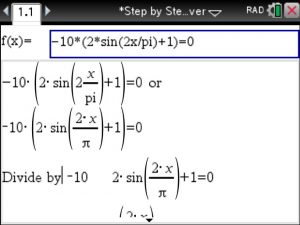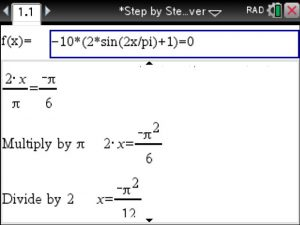Since we are solving a trigonometric (thus periodic) equation in above example
we obtain infinity many solutions whose setup is displayed in the
last line. n11 represents a constant, think of it as constant K as typically used in text books.
If you set n11 equal to 0 for the first expression for the solution x you recognize the solution
derived step by step.

Posted on Categories equation, solver, tinspirecx

## Step by Step Equation Solver – How to enter and solve some tricky equations

Say we need to solve :Since the Step by Step Equation Solver solves for the variable x we just replace v by x to enter as shown below: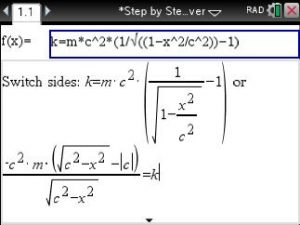Note: for the square root symbol press:  CTRL   +   x^2

Now view steps until the final answer is derived.

Posted on Categories solver, tips & tricks

## Video: Convert any Units – Step by Step – using the TINspire calculator

Your handy Ti-Nspire can now – step by step – convert any units such as time, mass, length, area, volume, speed, temperature, impulse, momentum, pressure, work, energy, density, pH-Level, Mass, Moles, degree/radian, DMS, Convert to any base, Polar coordinates etc

On top of that you can do prime checking, compute tips, gas consumption, currencies, etc and much more!!

Here is a brief video showing you how to convert units with ease:

Posted on Categories solver, units

## Solve ACT problems using the TI-Nspire CX software – Be prepared for the ACT exam

The most comprehensive ACT APP for TI Nspire CX calculators.

Users have boosted their ACT knowledge & scores.

Ideal for quick review in all ACT classes as well as classes involving Trigonometry, Statistics, Functions, Algebra, Matrices, Geometry, Complex Numbers, Sequences

Easy to use. Just plug in the equation or numbers and the correct answer shows.

Posted on Categories ACT, exam, solver

## Video: ACT app for the TI-NSpire CX (no CAS) calculator – Solve and Check ACT problems

Posted on Categories ACT, exam, solver